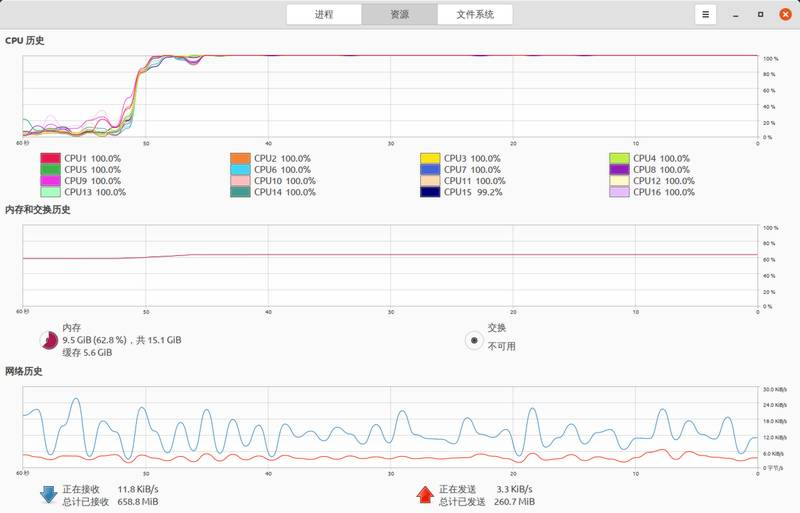首页 关于 笔记

## 前言1. 小明说：我不知道这两个数是多少。
2. 小红说：我也不知道这两个数是多少。
3. 小明说：我现在知道了。 请问这两个数到底是多少？

## 折腾

4和6都不是素数，都存在可能，答案明显不唯一

````package com.company;````
``````
````/**`` * 原题`` * 老师在纸上写了两个正整数，`` * 把它们的和告诉了小红，`` * 把它们的积告诉了小明。`` * 老师说：这两个正整数可能相等也可能不相等。`` * 1. 小明说：我不知道这两个数是多少。`` * 2. 小红说：我也不知道这两个数是多少。`` * 3. 小明说：我现在知道了。`` * 请问这两个数到底是多少？`` *`` * 思路`` * 结合 1，说明小明得到的数字 分解成 x * y 可能性不唯一`` * 结合 2，说明小红得到的数字 分解成 x + y 再结合成 x * y 结果依旧不唯一`` * 结合 3，说明小明得到的数字 分解成 x * y 再结合成 x + y 可以得到多个结果，但只有一个小红不能唯一，其余结果小红在2中必能解开`` */````
````public class Main {````
````    /**``     * 判断是否为素数``     */``    public static boolean isPrime(int number) {``        for (int i = 2; i < number; i++) {``            if (number % i != 0) {``                continue;``            }``            return false;``        }``        return true;``    }````
````    /**``     * 排除不合格的数字b``     * 拆解b 后模拟乘 不为素数的可能性必须大于2``     */``    public static boolean checkNumberB(int number) {``        int count = 0;``        for (int i = 1; i <= number / 2; i++) {``            int x = i;``            int y = number - i;``            if(!isPrime(x * y)){``                count ++;``            }``        }``        return count < 2;``    }````
````    /**``     * 能进入这里说明不是素数``     * 拆解a 后模拟加``     */``    public static boolean checkNumberA(int number) {``        int count = 0;``        for (int i = 1; i <= Math.sqrt(number); i++) {``            // 能整除``            if(number % i == 0){``                int x = i;``                int y = number / i;``                // 核心就是这句话 套娃``                if(checkNumberB(x + y)){``                    count ++;``                }``            }``        }``        // a这个数字拆分完 模拟完 只允许出现一个能通过的可能性 否则都不符合问题3``        return count != 1;``    }````
````    /**``     * 核心算法``     */``    public static void core() {``        for (int i = 1; i < 100; i++) {``            for (int j = 1; j < 100; j++) {``                int a = i * j;``                int b = i + j;``                // 对应问题1 a 不能是素数``                if (isPrime(a)) {``                    continue;``                }``                // 对应问题2 b 拆分后模拟 i*j所有可能性能通过验算``                if(checkNumberB(b)){``                    continue;``                }``                // 对应问题3 略``                if(checkNumberA(a)){``                    continue;``                }``                // 上面3个逻辑排除完 还没被排除的就是正确答案 i 和 j 就是那2个数，a 是积，b 是和``                System.out.println("i = " + i + " , j = " + j + " , a = " + a + " , b = " + b);``            }``        }``    }````
````    public static void main(String[] args) {``        core();``    }``}````

``i = 1 , j = 4 , a = 4 , b = 5``i = 4 , j = 1 , a = 4 , b = 5``

````package com.company;````
``````
````import java.util.concurrent.ExecutorService;``import java.util.concurrent.Executors;````
````/**`` * 原题`` * 老师在纸上写了两个正整数，`` * 把它们的和告诉了小红，`` * 把它们的积告诉了小明。`` * 老师说：这两个正整数可能相等也可能不相等。`` * 1. 小明说：我不知道这两个数是多少。`` * 2. 小红说：我也不知道这两个数是多少。`` * 3. 小明说：我现在知道了。`` * 请问这两个数到底是多少？`` * <p>`` * 思路`` * 结合 1，说明小明得到的数字 分解成 x * y 可能性不唯一`` * 结合 2，说明小红得到的数字 分解成 x + y 再结合成 x * y 结果依旧不唯一`` * 结合 3，说明小明得到的数字 分解成 x * y 再结合成 x + y 可以得到多个结果，但只有一个小红不能唯一，其余结果小红在2中必能解开`` */````
````public class Main {````
````    /**``     * 判断是否为素数``     */``    public static boolean isPrime(int number) {``        for (int i = 2; i < number; i++) {``            if (number % i != 0) {``                continue;``            }``            return false;``        }``        return true;``    }````
````    /**``     * 排除不合格的数字b``     * 拆解b 后模拟乘 不为素数的可能性必须大于2``     */``    public static boolean checkNumberB(int number) {``        int count = 0;``        for (int i = 1; i <= number / 2; i++) {``            int x = i;``            int y = number - i;``            if (!isPrime(x * y)) {``                count++;``            }``        }``        return count < 2;``    }````
````    /**``     * 能进入这里说明不是素数``     * 拆解a 后模拟加``     */``    public static boolean checkNumberA(int number) {``        int count = 0;``        for (int i = 1; i <= Math.sqrt(number); i++) {``            // 能整除``            if (number % i == 0) {``                int x = i;``                int y = number / i;``                // 核心就是这句话 套娃``                if (checkNumberB(x + y)) {``                    count++;``                }``            }``        }``        // 只能存在一个通过套娃 checkNumberB 的结果``        return count != 1;``    }````
````    /**``     * 核心算法``     */``    @SuppressWarnings("AlibabaThreadPoolCreation")``    public void core(int nThreads) {``        ExecutorService executorService = Executors.newFixedThreadPool(nThreads);``        for (int i = 1; i < 1000; i++) {``            for (int j = 1; j < 1000; j++) {``                executorService.submit(new CalcThread(i,j));````
````            }``        }``        executorService.shutdown();``    }````
``````
````    public static void main(String[] args) {``        // 开200个线程去跑``        new Main().core(200);``    }````
````    class CalcThread extends Thread {``        private int i;``        private int j;````
````        public CalcThread(int i , int j){``            this.i = i;``            this.j = j;``        }````
````        @Override``        public void run() {``            int a = i * j;``            int b = i + j;``            // 对应问题1 a 不能是素数``            if (isPrime(a)) {``                return;``            }``            // 对应问题2 b 拆分后模拟 i*j所有可能性能通过验算``            if (checkNumberB(b)) {``                return;``            }``            // 对应问题3 略``            if (checkNumberA(a)) {``                return;``            }``            // 上面3个逻辑排除完 还没被排除的就是正确答案 i 和 j 就是那2个数，a 是积，b 是和``            System.out.println("i = " + i + " , j = " + j + " , a = " + a + " , b = " + b);``        }``    }``}````

``i = 1 , j = 4 , a = 4 , b = 5``i = 4 , j = 1 , a = 4 , b = 5``## END{{comment.name}} {{comment.os}} {{comment.browser}}
{{dateFormatter(comment.createTime)}}

{{comment.message}}